# Geometry and Trigonometry

##The physical world in shape and space

So many applications of mathematics depend on understanding shape and space. This geometry and trigonometry module gets to the heart of that. For many, much of this will be a review of work you have done before with trigonometry, missing sides and missing angles, cirles and volume and surface area of 3d shapes. Where it might be new is with specific applications in and out of 3 dimensions. Also, dividing up space with Voronoi diagrams will be new to most!

## What is in this section?

#### 3.1 Volume and surface area

This section is all about the volume and surface area of prisms, cylinders, pyramids, cones and spheres! All of these shapes has their own unique properties and this section is about understanding and calculating the volumes and surface areas.

#### 3.2 Right angled Trigonometry

This part of trigonometry can be seen in the recurring relationships between the sides of right angles triangles. The relationship means that we can use two bits of information about a triangle to work out a third and so on until we know everything...

#### 3.2 Non-right angled trigonometry

Here we take trigonometry to the next level by working with triangles that do not have a right angle. We will work on three key rules. The Sine rule, the Cosine rule and the formula for th area of a triangle. All of these involve linking four...

#### 3.3 Applications of trigonometry

Given the focus on applications in this course, we will look more specifically at real world problems which trigonometry can help solve. Examples of this include bearings and angles of elevation/depression, which are likely to form a large...

#### 3.4 Circles

Circles In this section you will learn how to find the length of an arc of a circle and the area of a sector. Make sure you are confident rearranging formulae and solving equations before making a start on this page! Key Concepts In this unit...

#### 3.5 & 3.6 Perpendicular Bisectors & Voronoi Diagrams

In this fascinating topic, we will delve into the wonderful world of Voronoi diagrams. This includes creating Voronoi diagrams with perpendicular bisectors, adding a new site, nearest neighbour interpolation and the toxic waste dump problem....

## Key Questions

The following are list of key questions that you might be asking about these topics with links to the places where you can get the answers!

## How do we calculate with area and circumference of a circle?

There are some useful formulae to learn how to use to help you to do this:    3.4 Circles

## How do you find the area of a sector and the length of an arc of a circle?

We can look at the fractions of the circle that we are trying to find in order to do this.   3.4 Circles

## What are Voronoi diagrams used for?

Voronoi diagrams have a huge number of applications, from city planning, to architecture, to biological modelling.    3.5 & 3.6 Perpendicular Bisectors & Voronoi Diagrams

## How can we find a missing angle or length of a right angled triangle?

Trigonometry can be used to find this.   3.2 Right angled Trigonometry

## How are volume and surface area calculated?

We can use various formulae to help us to find volume and surface area   3.1 Volume and surface area

•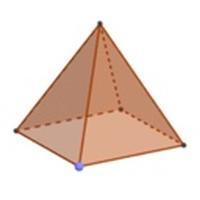#### 3.1 Volume and surface area

This section is all about the volume and surface area of prisms, cylinders, pyramids, cones and spheres! All of these shapes has their own unique properties and this section is about understanding and calculating the volumes and surface areas.

•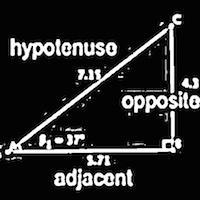#### 3.2 Right angled Trigonometry

This part of trigonometry can be seen in the recurring relationships between the sides of right angles triangles. The relationship means that we can use two bits of information about a triangle to work out a third and so on until we know everything...

•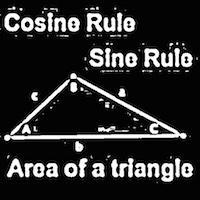#### 3.2 Non-right angled trigonometry

Here we take trigonometry to the next level by working with triangles that do not have a right angle. We will work on three key rules. The Sine rule, the Cosine rule and the formula for th area of a triangle. All of these involve linking four...

•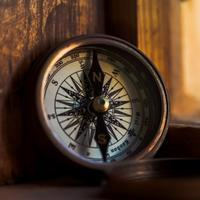#### 3.3 Applications of trigonometry

Given the focus on applications in this course, we will look more specifically at real world problems which trigonometry can help solve. Examples of this include bearings and angles of elevation/depression, which are likely to form a large...

•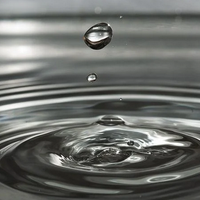#### 3.4 Circles

Circles In this section you will learn how to find the length of an arc of a circle and the area of a sector.   Keep track of your progress and practice the exam questions using this document: Check that you are confident learnin Can you round...

•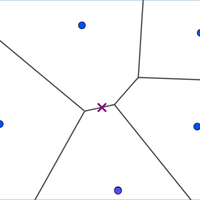#### 3.5 & 3.6 Perpendicular Bisectors & Voronoi Diagrams

In this fascinating topic, we will delve into the wonderful world of Voronoi diagrams. This includes creating Voronoi diagrams with perpendicular bisectors, adding a new site, nearest neighbour interpolation and the toxic waste dump problem....